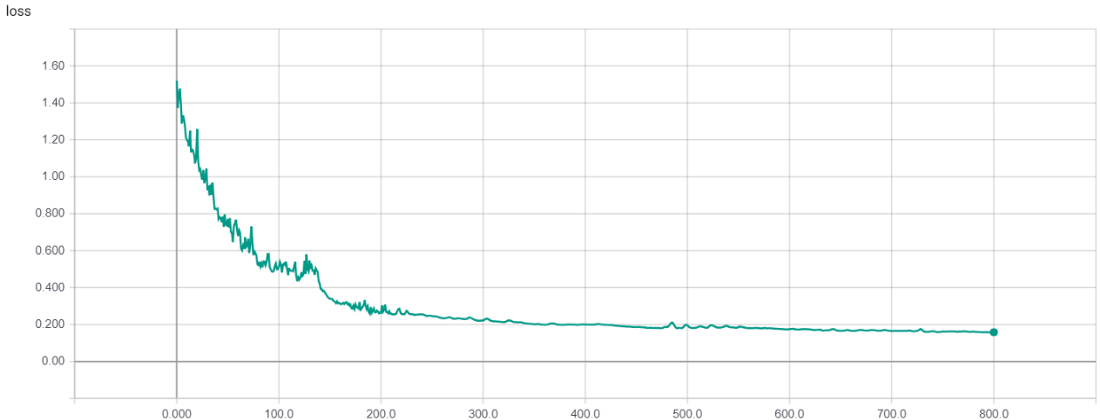Training Recurrent Neural Networks on Long Sequences

Deep Recurrent Neural Networks (RNN) are a type of Artificial Neural Network that takes the networks previous hidden state as part of its input, effectively allowing the network to have a memory. This makes RNNs useful for modeling sequential or time-series data such as stock prices. However, training RNNs on sequences greater than a few hundred time steps can be difficult. In this post, we will explore three tools that can allow for more efficient training of RNN models with long sequences: Optimizers, Gradient Clipping, and Batch Sequence Length.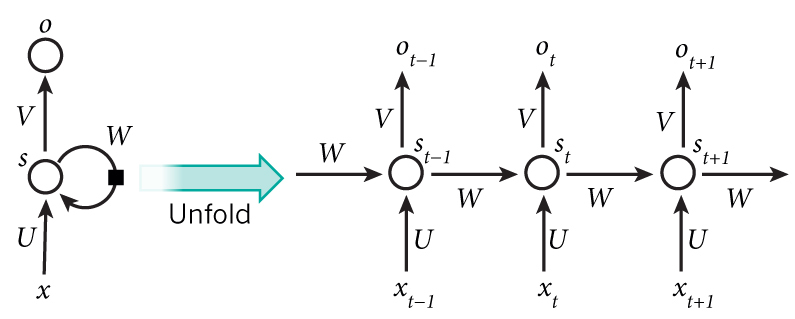Recurrent Neural Network Cell. Source: Nature

Creating Our Training Data

For the purposes of this post, the training data used is not particularly important since we are solely looking at methods for improving model fitting on training data. That being said, I will present the method purposed for generating the data used in the following experiments.

Non-stationary, single-valued data can be generated by adding together two sinusoidal waves with fixed frequency and stochastically varying amplitudes. The amplitudes are determined by the Cartesian coordinates of a unit vector, tracing a unit circle with slow, random changes of angle theta around the origin. Gaussian noise with variance 0.01 is added to the generated data and a bias of 100 is added to make all values positive.

```import numpy as np
```
```#function for getting coordinates given an angledef get_cartesian_coords(nums):theta = numsx = np.cos(theta)y = np.sin(theta)return [x,y]
```
```#generate random starting coordinateangle = np.random.uniform(-np.pi, np.pi)coordinates = get_cartesian_coords(random_angle)amplitudes = [abs(coord) for coord in coordinates]random_walk = [amplitudes]
```
```for _ in range(19999):#generate random small change in angledirection = 0.01 * np.random.uniform(-1, 1)angle += directioncoordinates = get_cartesian_coords(angle)amplitudes = [abs(coord) for coord in coordinates]random_walk.append(amplitudes)
```
```timestep = list(range(20000))#create sequence using generated amplitudessequence = list(map(lambda i: np.round((((random_walk[i])*-1)*np.sin((0.0002)*i)) + ((random_walk[i])*np.sin(0.0002*i)))+ 100 + np.random.normal(0, 0.01),decimals=2),timestep)
```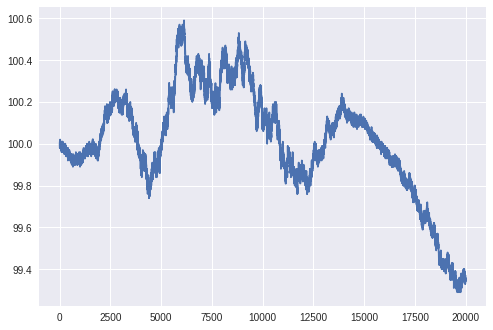Sample Training Data Sequence

The generated data will be treated as if it were Price data for a commodity that we can buy or sell. Our model will learn to output the future returns of buying or selling the commodity at each time-step. The training data outputs are calculated using Bellman’s Q-Value equation as discussed by Gayatri Vadali in her post Q Matrix Update to train Deep Recurrent Q Networks More Effectively.

15 sequences, each with a length of 20000 time-steps, were generated to be trained on the RNN network. The model will have to learn the underlying constituent modes that make up the data and learn to detect which mode has the dominant amplitude at each given time-step in order to to predict future values.

Model

We will use Long-short Term Memory Cells (LSTM) as the RNN layers in our model. The network architecture consists of two feed-forward layers followed by two LSTM layers. Since regularization is typically used during training, we will include random noise layers between each Dense and LSTM layer for regularization. The input data to our model is the change in value from the previous 128 time steps, giving us 128 individual input features. Here is the model summary in Keras: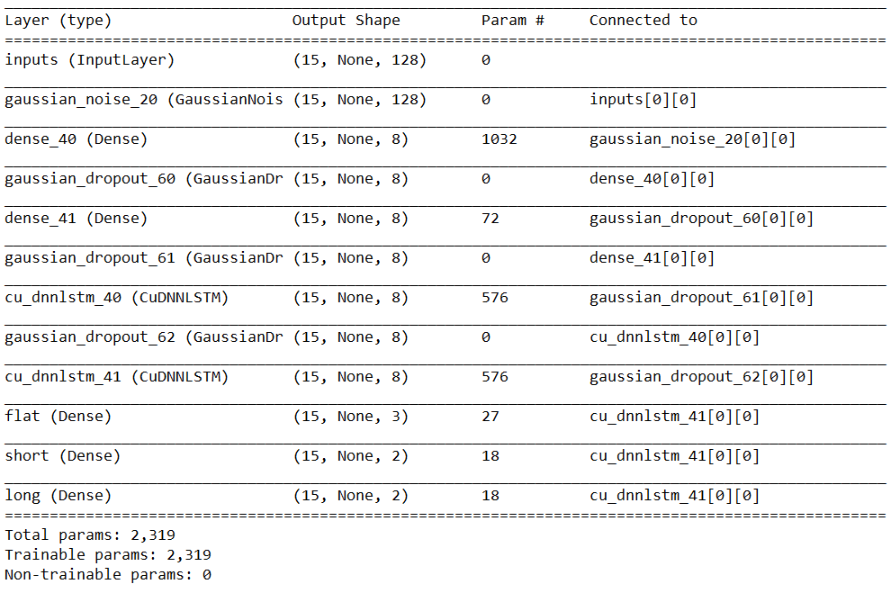Training

As a baseline, the model is trained using Stochastic Gradient Descent with a learning rate of 0.01. Our training batch consists of all 15 sequences together. Keras requires that all sequences in a batch are of identical length. In this case, all sequences are 20000 time-steps. If we had varying sequence length, we would need to zero pad our data so that it could fit into a numpy array, as required by Keras.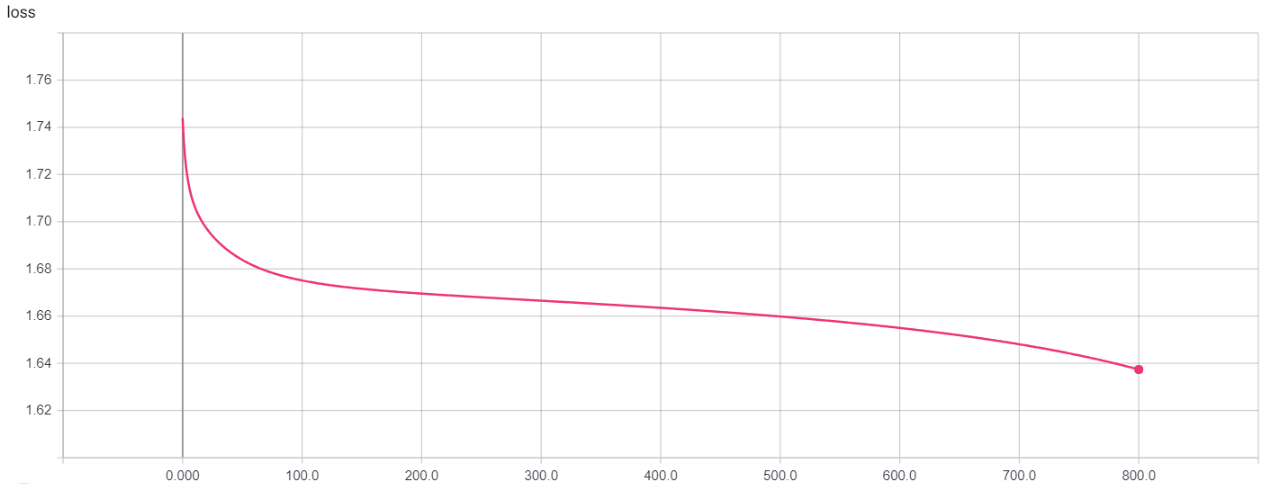Stochastic Gradient Descent, Learning Rate = 0.01

Lets see how we can improve training. We will explore three areas: Optimizers, Gradient Clipping, and Batch Sequence Length.

Optimizers

Two commonly used variations of Stochastic Gradient Descent (SGD) are RMSprop and Adam. While SGD makes weight and bias updates independently, RMSprop makes its updates based upon the root mean square of the differentials of weights and biases, allowing a straighter trajectory towards the optimal loss value. The Adam optimizer works similar to RMSprop but includes a moving average of the second order moment of gradients for an adaptive learning rate.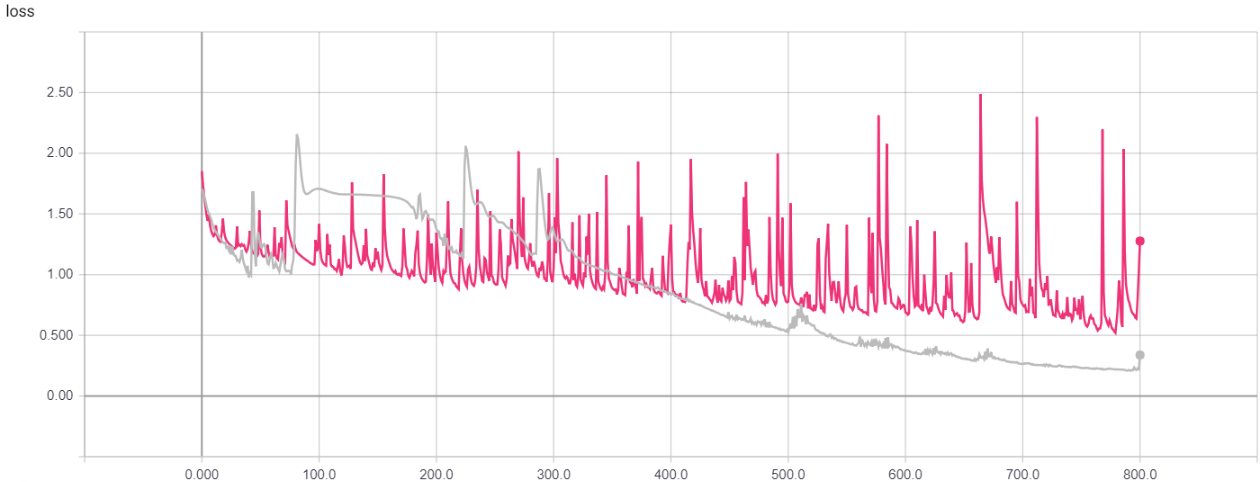Red: [RMSprop, Learning Rate = 0.01], Gray: [Adam, Learning Rate = 0.01]

Both optimizers are a significant improvement over SGD. Adam performs better than RMSprop, but there are many large spikes in loss that stalled the training process. We will look at a way of removing these spikes in the next section.

Clip Norm

Neural network optimizers rely on gradient descent for determining model weight updates. During training, weight updates are evaluated by backpropagating gradients through all layers of the network via multiplication. In a Recurrent Neural Network, gradients are backpropogated through all time steps as well. This means that the longer our sequence size is, the more gradients we will be taking the product of. Even a single gradient that is either extremely small or extremely large can dominate our weight updates. A very small gradient is known as a vanishing gradient, which results in the model failing to make any further significant weight updates. A very large gradient is known as an exploding gradient, which leads to highly unstable weight updates and loss values.

Vanishing gradients are mostly avoided through the use of LSTM cells. LSTMs have a hidden state which allows gradients to pass straight through all time-steps of the network. Exploding gradients, however, are still a problem for long sequences. In our previous training attempt, exploding gradients caused massive spikes in loss and took the network on a few detours before finally converging. To combat exploding gradients, we will ‘clip’ any gradient that has a value larger than unit normal from the average. This way, we will catch any sudden explosion in our weight updates, keeping training much more stable.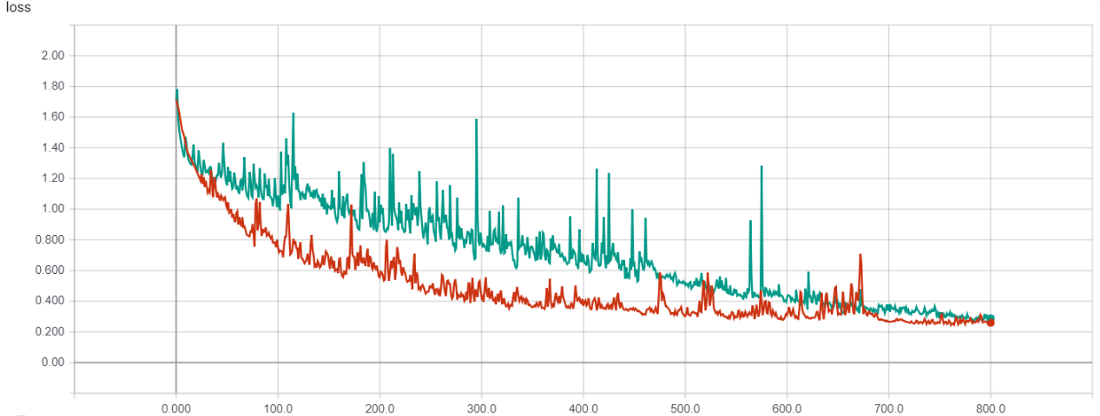Gradient clipping removes the large spikes in loss and greatly improves training stability. Adam maintains the advantage over RMSprop.

Batch Sequence Length

Instead of using the entirety of our sequences during each training batch, let’s break up our sequences into smaller portions and train on batches of one portion of each sequence at a time. For example, let’s consider a batch sequence length of 100. We divide each of our original 20000 time-step sequences into 100 sub-sequences, each of length 200. When we train our network, our first batch contains the first 200 time-steps of each sequence. Our second batch contains the next 200 time-steps of each sequence, and so on. It is vital to preserve the order of the sequences so that the hidden state of each sequence can be propagated from one batch to the next. In this experiment, we start with a batch sequence length of 100. Once the loss value fails to decrease for 20 straight epochs, we use a batch sequence length of 50, then 10, 2, and finally back to 1, the full sequence. For testing this method, we revert back to the Stochastic Gradient Descent Optimizer with no gradient clipping in order to compare against our original benchmark.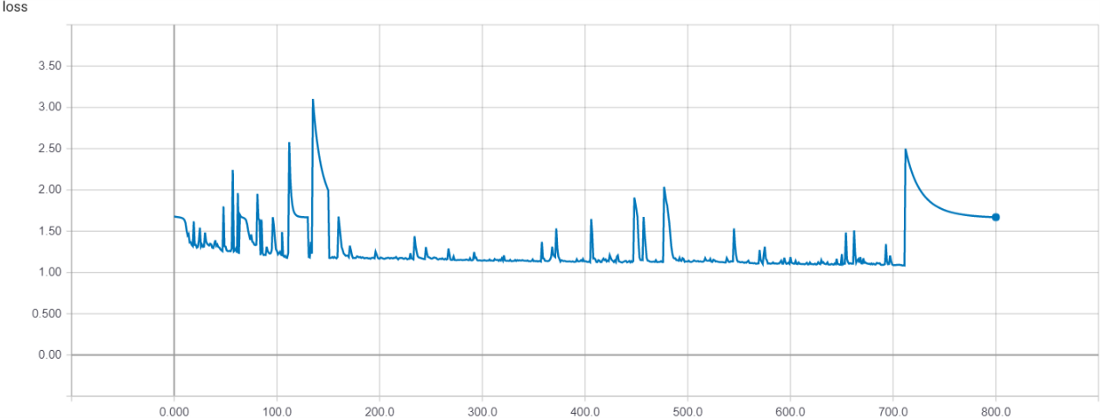Training on smaller sequences provides a significant initial speed up in loss reduction. Now let’s try using smaller batch sequence lengths in conjunction with Adam optimizer and gradient clipping.